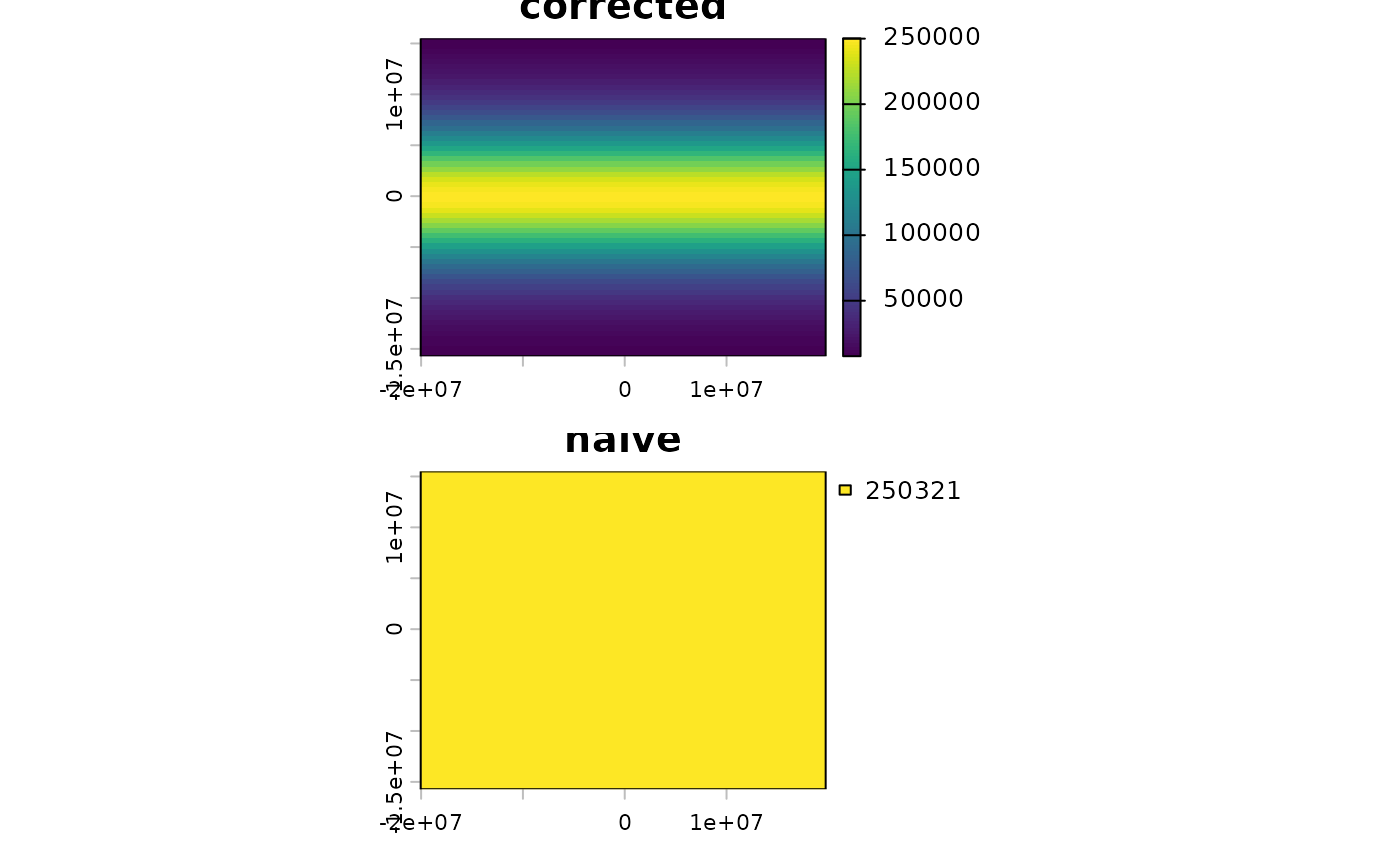Compute the area covered by individual raster cells.

Computing the surface area of raster cells is especially relevant for longitude/latitude rasters.

But note that for both angular (longitude/latitude) and for planar (projected) coordinate reference systems raster cells sizes are generally not constant, unless you are using an equal-area coordinate reference system.

For planar CRSs, the area is therefore not computed based on the linear units of the coordinate reference system, but on the *actual* area by transforming cells to longitude/latitude. If you do not want that correction, you can use transform=FALSE or init(x, prod(res(x)))

# S4 method for SpatRaster
cellSize(x, mask=FALSE, lyrs=FALSE, unit="m", transform=TRUE, rcx=100, filename="", ...)

## Arguments

x

SpatRaster

logical. If TRUE, cells that are NA in x are also NA in the output

lyrs

logical. If TRUE and mask=TRUE, the output has the same number of layers as x. That is only useful if cases where the layers of x have different cells that are NA

unit

character. One of "m", "km", or "ha"

transform

logical. If TRUE, planar CRS data are transformed to lon/lat for accuracy

rcx

positive integer. The maximum number of rows and columns to be used to compute area of planar data if transform=TRUE. If x has more rows and/or columns, the raster is aggregated to match this limit, and values for the original cells are estimated by bilinear interpolation (see resample). This can save a lot of time

filename

character. Output filename

...

additional arguments for writing files as in writeRaster

## Value

SpatRaster

expanse

## Examples


# SpatRaster
r <- rast(nrows=18, ncols=36)
v <- 1:ncell(r)
v[200:400] <- NA
values(r) <- v

# size of each raster cell
a <- cellSize(r)

# illustration of distortion
r <- rast(ncols=90, nrows=45, ymin=-80, ymax=80)
m <- project(r, "+proj=merc")

bad <- init(m, prod(res(m)) / 1000000, names="naive")
good <- cellSize(m, unit="km", names="corrected")